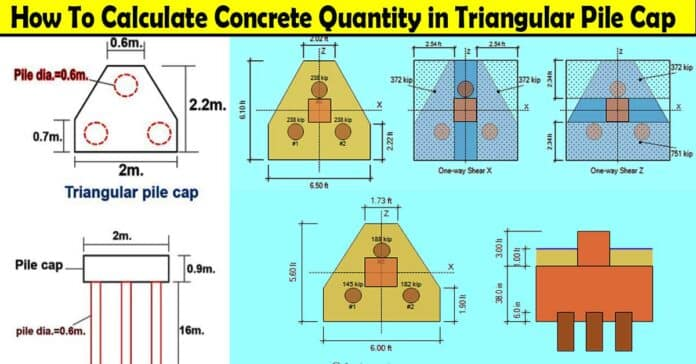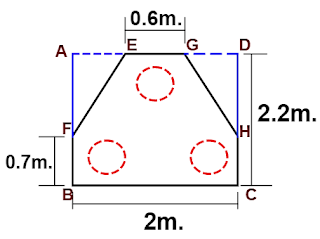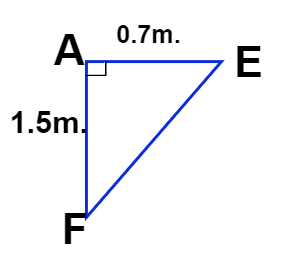## How to Calculate Volume of Concrete in a Triangular Pile Cap – 3 Piles

In this Article today we will talk about the Volume of Concrete in Triangular Pile | Pile Concrete Calculation Formula | Triangular Pile Cap Volume | Piles Foundation Types | Concrete Piling | Concrete Piles Construction | Concrete Quantity of Pile | Pile Concrete Calculator | Foundation Pilings

## What is Pile Cap?

Pile cap is a structure which is built when single pile will not be able to take the load of the overlying structure

This is generally built when there are some restrictions on the size of a single pile

Pile cap is like a slab below which there are various piles or a group of piles . Such that they combine to act as a single pile which witstand the load from the superstructure or column. Concrete Piling

## How to Find Volume of Concrete in a Triangular Pile Cap?

Let us calculate the volume of concrete in a triangular pile foundation having 3 nos. of the pile, as shown below. Foundation Pilings

### Given data:

Pile diameter = 0.6m.( d )

No. of piles = 3 nos.

Length of pile = 16m. (h )

Depth of the pile cap = 0.9m.(D )

### Solution:

The volume of concrete in the piles

= 3nos.× πr2 h

Here,

r = radius of the pile.

= d ÷ 2

= 0.6m ÷ 2

= 0.3m.

h = length of the pile.

The volume of concrete in piles

= 3nos. × 3.142 × (0.3m)2 × 16m.

13.57 cum.

The volume of concrete in the pile cap :

The volume of  pile cap concrete

=  Surface area (A ) × depth ( D )

First, let us calculate the sectional area (A1) of rectangle ABCD, as shown in the below drawing.Area of rectangle ABCD (A1)

=L × B

= 2.2m × 2.0m

= 4.4 sqm.

To get the surface area ( A) of the pile cap, we have to deduct the area of triangles AEF & GDH from the rectangle area.

Here,

Triangle AEF = triangle GDH

Area of triangle AEF

= 0.5 × base × height.

= 0.5 × side AE × side AF

Side AE

= [ ( 1/2 ×side BC ) – (1/2 × side EG )]

=  [ ( 1/2 ×2m. ) – (1/2 × 0.6m. )]

= [ 1m – 0.3 m ]

0.7m.

Side AF

= side AB – side FB

= 2.2m – 0.7m

1.5m.

I have redrawn the triangle, with the calculated length of the sides AF & AE as shown below.Area of triangle AEF

= 0.5 × side AE × side AF
= 0.5 × 0.7m × 1.5m.
0.525 sqm.

The surface area of the pile cap (A )
= [area of rectangle ABCD – (2nos. × area of a triangle AEF )]
= [4.4 sqm. – (2nos. × 0.525sqm.)]
= [4.4 sqm. – 1.05 sqm.]
= 3.35 sqm.

Now, the concrete vol. of pile cap
= surface area (A ) × depth (D )
= 3.35 sqm. × 0.9m.
3.015 cum.

The total concrete volume of 3-pile foundation

= The vol. of concrete in pile cap + total vol. of concrete in piles.
= 3.015 cum + 13.57 cum.
16.585 cum.

## Conclusion:

Full article on Volume of Concrete in Triangular Pile | Pile Concrete Calculation Formula | Triangular Pile Cap Volume | Piles Foundation Types | Concrete Piling | Concrete Piles Construction | Concrete Quantity of Pile | Pile Concrete Calculator | Foundation Pilings. Thank you for the full reading of this article in “The Civil Engineering” platform in English. If you find this post helpful, then help others by sharing it on social media. If any formula of BBS is missing from this article please tell me in comments.

1.px